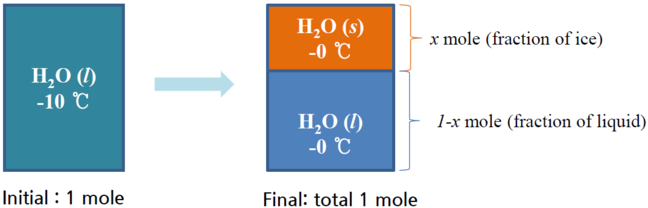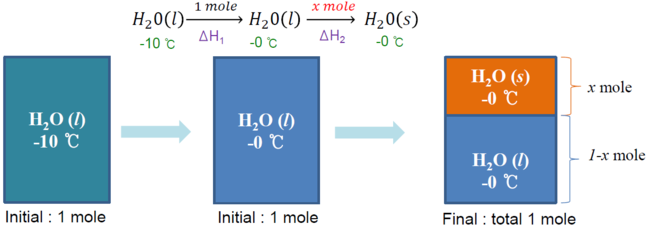## Want to keep learning?

This content is taken from the Hanyang University's online course, Thermodynamics in Energy Engineering. Join the course to learn more.
2.4

# Example 1 : supercooled water

If the system is insulated (adiabatic), -10℃ H$${2}$$O$$(l)$$ (supercooled liquid) will be transformed to ice + liquid H$${2}$$O at 0℃. Calculate fraction of ice in the final mixture.

Basis : 1 mole of initial supercooled liquid.• Since the system is adiabatic, Qp=ΔHtotal=0.
• In calculating enthalpy changes, take the reversible path. The enthalpy change of reversible path is the same with that of the original change since enthalpy is a state function.
• Regard the process as follows.